# comm.DecisionFeedbackEqualizer

Equalize modulated signals using decision feedback filtering

Since R2019a

## Description

The `comm.DecisionFeedbackEqualizer` System object™ uses a decision feedback filter tap delay line with a weighted sum to equalize modulated signals transmitted through a dispersive channel. The equalizer object adaptively adjusts tap weights based on the selected algorithm. For more information, see Algorithms.

To equalize modulated signals using a decision feedback filter:

1. Create the `comm.DecisionFeedbackEqualizer` object and set its properties.

2. Call the object with arguments, as if it were a function.

## Creation

### Syntax

``dfe = comm.DecisionFeedbackEqualizer``
``dfe = comm.DecisionFeedbackEqualizer(Name,Value)``

### Description

example

````dfe = comm.DecisionFeedbackEqualizer` creates a decision feedback equalizer System object to adaptively equalize a signal.```

example

````dfe = comm.DecisionFeedbackEqualizer(Name,Value)` sets properties using one or more name-value pairs. For example, `comm.DecisionFeedbackEqualizer('Algorithm','RLS')` configures the equalizer object to update tap weights using the recursive least squares (RLS) algorithm. Enclose each property name in quotes.```

## Properties

expand all

Unless otherwise indicated, properties are nontunable, which means you cannot change their values after calling the object. Objects lock when you call them, and the `release` function unlocks them.

If a property is tunable, you can change its value at any time.

Adaptive algorithm used for equalization, specified as one of these values:

Data Types: `char` | `string`

Number of forward equalizer taps, specified as a positive integer. The number of forward equalizer taps must be greater than or equal to the value of the `InputSamplesPerSymbol` property.

Data Types: `double`

Number of feedback equalizer taps, specified as a positive integer.

Data Types: `double`

Step size used by the adaptive algorithm, specified as a positive scalar. Increasing the step size reduces the equalizer convergence time but causes the equalizer output estimates to be less stable.

Tip

To determine the maximum step size allowed, use the `maxstep` object function.

Tunable: Yes

#### Dependencies

To enable this property, set Algorithm to `'LMS'` or `'CMA'`.

Data Types: `double`

Forgetting factor used by the adaptive algorithm, specified as a scalar in the range (0, 1]. Decreasing the forgetting factor reduces the equalizer convergence time but causes the equalizer output estimates to be less stable.

Tunable: Yes

#### Dependencies

To enable this property, set Algorithm to `'RLS'`.

Data Types: `double`

Initial inverse correlation matrix, specified as a scalar or an NTaps-by-NTaps matrix. NTaps is equal to the sum of the NumForwardTaps and NumFeedbackTaps property values. If you specify `InitialInverseCorrelationMatrix` as a scalar, a, the equalizer sets the initial inverse correlation matrix to a times the identity matrix: a(`eye`(NTaps)).

#### Dependencies

To enable this property, set Algorithm to `'RLS'`.

Data Types: `double`

Signal constellation, specified as a vector. The default value is a QPSK constellation generated using this code: `pskmod(0:3,4,pi/4)`.

Data Types: `double`

Reference tap, specified as a positive integer less than or equal to the NumForwardTaps property value. The equalizer uses the reference tap location to track the main energy of the channel.

Data Types: `double`

Input signal delay in samples relative to the reset time of the equalizer, specified as a nonnegative integer. If the input signal is a vector of length greater than 1, then the input delay is relative to the start of the input vector. If the input signal is a scalar, then the input delay is relative to the first call of the System object and to the first call of the System object after calling the `release` or `reset` object function.

Data Types: `double`

Number of input samples per symbol, specified as a positive integer. Setting this property to any number greater than one effectively creates a fractionally spaced equalizer.

Data Types: `double`

Enable training control input, specified as a logical `0` (`false`) or `1` (`true`). Setting this property to `true` enables the equalizer training flag input `tf`.

Data Types: `logical`

Update tap weights when not training, specified as a logical `1` (`true`) or `0` (`false`). If this property is set to `true`, the System object uses decision directed mode to update equalizer tap weights. If this property is set to `false`, the System object keeps the equalizer tap weights unchanged after training.

Data Types: `logical`

Source of adapt tap weights request, specified as one of these values:

• `'Property'` — Specify this value to use the AdaptWeights property to control when the System object adapts tap weights.

• `'Input port'` — Specify this value to use the `aw` input to control when the System object adapts tap weights.

#### Dependencies

To enable this property, set Algorithm to `'CMA'`.

Data Types: `char` | `string`

Adapt tap weights, specified as a logical `1` (`true`) or `0` (`false`). If this property is set to `true`, the System object updates the equalizer tap weights. If this property is set to `false`, the System object keeps the equalizer tap weights unchanged.

#### Dependencies

To enable this property, set AdaptWeightsSource to `'Property'` and set AdaptAfterTraining to `true`.

Data Types: `logical`

Source for initial tap weights, specified as one of these values:

• `'Auto'` — Initialize the tap weights to the algorithm-specific default values, as described in the InitialWeights property.

• `'Property'` — Initialize the tap weights using the InitialWeights property value.

Data Types: `char` | `string`

Initial weights used by the adaptive algorithm, specified as a scalar or vector. The default is `0` when the Algorithm property is set to `'LMS'` or `'RLS'`. The default is `[0;0;1;0;0]` when the Algorithm property is set to `'CMA'`.

If you specify `InitialWeights` as a scalar, the equalizer uses scalar expansion to create a vector of length NTaps with all values set to `InitialWeights`. NTaps is equal to the sum of the NumForwardTaps and NumFeedbackTaps property values. If you specify `InitialWeights` as a vector, the vector length must be NTaps.

Data Types: `double`

Tap weight update period in symbols, specified as a positive integer. The equalizer updates the tap weights after processing this number of symbols.

Data Types: `double`

## Usage

### Syntax

``y = dfe(x,tsym)``
``y = dfe(x,tsym,tf)``
``y = dfe(x)``
``y = dfe(x,aw)``
``[y,err] = dfe(___)``
``[y,err,weights] = dfe(___)``

### Description

example

````y = dfe(x,tsym)` equalizes input signal `x` by using training symbols `tsym`. The output is the equalized symbols. To enable this syntax, set the Algorithm property to `'LMS'` or `'RLS'`.```

example

````y = dfe(x,tsym,tf)` also specifies training flag `tf`. The System object starts training when `tf` changes from `false` to `true` (at the rising edge). The System object trains until all symbols in `tsym` are processed. The input `tsym` is ignored when `tf` is `false`. To enable this syntax, set the Algorithm property to `'LMS'` or `'RLS'` and TrainingFlagInputPort property to `true`.```
````y = dfe(x)` equalizes input signal `x`. To enable this syntax, set the Algorithm property to `'CMA'`.```

example

````y = dfe(x,aw)` also specifies adapts weights flag `aw`. If `aw` is `true`, the System object adapts the equalizer tap weights. If `aw` is `false`, the System object keeps the weights unchanged. To enable this syntax, set the Algorithm property to `'CMA'` and AdaptWeightsSource property to `'Input port'`.```
````[y,err] = dfe(___)` also returns error signal `err` using input arguments from any of the previous syntaxes.```

example

````[y,err,weights] = dfe(___)` also returns `weights`, the tap weights from the last tap weight update, using input arguments from any of the previous syntaxes.```

### Input Arguments

expand all

Input signal, specified as a column vector. The input signal vector length must be equal to an integer multiple of the InputSamplesPerSymbol property value. For more information, see Symbol Tap Spacing.

Data Types: `double`
Complex Number Support: Yes

Training symbols, specified as a column vector of length less than or equal to the length of input `x`. The input `tsym` is ignored when `tf` is `false`.

#### Dependencies

To enable this argument, set the Algorithm property to `'LMS'` or `'RLS'`.

Data Types: `double`

Training flag, specified as a logical `1` (`true`) or `0` (`false`). The System object starts training when `tf` changes from `false` to `true` (at the rising edge). The System object trains until all symbols in `tsym` are processed. The input `tsym` is ignored when `tf` is `false`.

#### Dependencies

To enable this argument, set the Algorithm property to `'LMS'` or `'RLS'` and TrainingFlagInputPort property to `true`.

Data Types: `logical`

Adapt weights flag, specified as a logical `1` (`true`) or `0` (`false`). If `aw` is `true`, the System object adapts weights. If `aw` is `false`, the System object keeps the weights unchanged.

#### Dependencies

To enable this argument, set the Algorithm property to `'CMA'` and AdaptWeightsSource property to `'Input port'`.

Data Types: `logical`

### Output Arguments

expand all

Equalized symbols, returned as a column vector that has the same length as input signal `x`.

Error signal, returned as a column vector that has the same length as input signal `x`.

Tap weights, returned as a column vector that has NTaps elements. NTaps is equal to the sum of the NumForwardTaps and NumFeedbackTaps property values. `weights` contains the tap weights from the last tap weight update.

## Object Functions

To use an object function, specify the System object as the first input argument. For example, to release system resources of a System object named `obj`, use this syntax:

`release(obj)`

expand all

 `isLocked` Determine if System object is in use `clone` Create duplicate System object `info` Characteristic information about the equalizer object `maxstep` Maximum step size for LMS equalizer convergence `mmseweights` Linear equalizer MMSE tap weights
 `step` Run System object algorithm `release` Release resources and allow changes to System object property values and input characteristics `reset` Reset internal states of System object

## Examples

collapse all

Create a BPSK modulator and an equalizer System object™, specifying a decision feedback LMS equalizer having eight forward taps, five feedback taps, and a step size of 0.03.

```bpsk = comm.BPSKModulator; eqdfe_lms = comm.DecisionFeedbackEqualizer('Algorithm','LMS', ... 'NumForwardTaps',8,'NumFeedbackTaps',5,'StepSize',0.03);```

Change the reference tap index of the equalizer.

`eqdfe_lms.ReferenceTap = 4;`

Build a set of test data. Receive the data by convolving the signal.

```x = bpsk(randi([0 1],1000,1)); rxsig = conv(x,[1 0.8 0.3]);```

Use `maxstep` to find the maximum permitted step size.

`mxStep = maxstep(eqdfe_lms,rxsig)`
```mxStep = 0.1028 ```

Equalize the received signal. Use the first 200 symbols as the training sequence.

`y = eqdfe_lms(rxsig,x(1:200));`

Apply decision feedback equalization using the least mean squares (LMS) algorithm to recover QPSK symbols passed through a delayed multipath AWGN channel.

Initialize simulation variables.

```M = 4; % QPSK numSymbols = 10000; numTrainingSymbols = 1000; chtaps = [1 0.5*exp(1i*pi/6) 0.1*exp(-1i*pi/8)];```

Generate QPSK-modulated symbols. Apply delayed multipath channel filtering and AWGN impairments to the symbols.

```data = randi([0 M-1], numSymbols, 1); tx = pskmod(data, M, pi/4); rx = awgn(filter(chtaps,1,tx),25,'measured');```

Create a decision feedback equalizer System object and display the default configuration. Adjust the reference tap to `1`. Check the maximum permitted step size. Equalize the impaired symbols.

`eq = comm.DecisionFeedbackEqualizer`
```eq = comm.DecisionFeedbackEqualizer with properties: Algorithm: 'LMS' NumForwardTaps: 5 NumFeedbackTaps: 3 StepSize: 0.0100 Constellation: [0.7071 + 0.7071i -0.7071 + 0.7071i -0.7071 - 0.7071i 0.7071 - 0.7071i] ReferenceTap: 3 InputDelay: 0 InputSamplesPerSymbol: 1 TrainingFlagInputPort: false AdaptAfterTraining: true InitialWeightsSource: 'Auto' WeightUpdatePeriod: 1 ```
```eq.ReferenceTap = 1; mxStep = maxstep(eq,rx)```
```mxStep = 0.2149 ```
`[y,err,weights] = eq(rx,tx(1:numTrainingSymbols));`

Plot the constellation of the impaired and equalized symbols.

```constell = comm.ConstellationDiagram('NumInputPorts',2); constell(rx,y)```Plot the equalizer error signal and compute the error vector magnitude of the equalized symbols.

```plot(abs(err)) grid on; xlabel('Symbols'); ylabel('|e|')```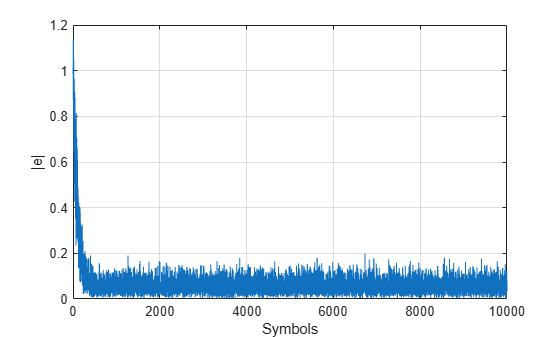```errevm = comm.EVM; evm = errevm(tx,y)```
```evm = 10.1288 ```

Plot the equalizer tap weights.

```subplot(3,1,1); stem(real(weights)); ylabel('real(weights)'); xlabel('Tap'); grid on; axis([1 8 -0.5 1]) line([eq.NumForwardTaps+0.5 eq.NumForwardTaps+0.5], ... [-0.5 1],'Color','r','LineWidth',1) title('Equalizer Tap Weights') subplot(3,1,2); stem(imag(weights)); ylabel('imag(weights)'); xlabel('Tap'); grid on; axis([1 8 -0.5 1]) line([eq.NumForwardTaps+0.5 eq.NumForwardTaps+0.5], ... [-0.5 1],'Color','r','LineWidth',1) subplot(3,1,3); stem(abs(weights)); ylabel('abs(weights)'); xlabel('Tap'); grid on; axis([1 8 -0.5 1]) line([eq.NumForwardTaps+0.5 eq.NumForwardTaps+0.5], ... [-0.5 1],'Color','r','LineWidth',1)```Demonstrate decision feedback equalization using the least mean squares (LMS) algorithm to recover QPSK symbols passed through an AWGN channel. Apply different equalizer training schemes and show the symbol error magnitude.

System Setup

Simulate a QPSK-modulated system subject to AWGN. Transmit packets composed of 200 training symbols and 1800 random data symbols. Configure a decision feedback LMS equalizer to recover the packet data.

```M = 4; numTrainSymbols = 200; numDataSymbols = 1800; SNR = 20; trainingSymbols = ... pskmod(randi([0 M-1],numTrainSymbols,1),M,pi/4); numPkts = 10; dfeq = comm.DecisionFeedbackEqualizer( ... Algorithm="LMS", ... NumForwardTaps=5, ... NumFeedbackTaps=4, ... ReferenceTap=3, ... StepSize=0.01);```

Train the Equalizer at the Beginning of Each Packet with Reset

Process each packet using prepended training symbols. Reset the equalizer after processing each packet. Resetting the equalizer after each packet forces the equalizer to train taps with no a priori knowledge. Equalizer error signal plots for the first, second, and last packet show higher symbol errors at the start of each packet.

```jj = 1; figure for ii = 1:numPkts b = randi([0 M-1],numDataSymbols,1); dataSym = pskmod(b,M,pi/4); packet = [trainingSymbols;dataSym]; rx = awgn(packet,SNR); [~,err] = dfeq(rx,trainingSymbols); reset(dfeq) if (ii ==1 || ii == 2 ||ii == numPkts) subplot(3,1,jj) plot(abs(err)) ylim([0 1]) title(['Packet # ',num2str(ii)]) xlabel('Symbols'); ylabel('Error Magnitude'); grid on; jj = jj+1; end end```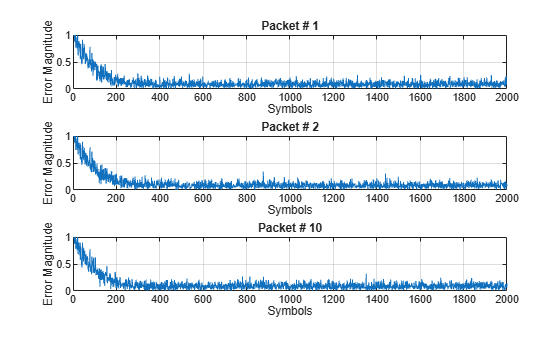Train the Equalizer at the Beginning of Each Packet Without Reset

Process each packet using prepended training symbols. Do not reset the equalizer after each packet is processed. By not resetting after each packet, the equalizer retains tap weights from training prior packets. Equalizer error signal plots for the first, second, and last packet show that after the initial training on the first packet, subsequent packets have less symbol errors at the start of each packet.

```release(dfeq) jj = 1; figure for ii = 1:numPkts b = randi([0 M-1],numDataSymbols,1); dataSym = pskmod(b,M,pi/4); packet = [trainingSymbols;dataSym]; channel = 1; rx = awgn(packet*channel,SNR); [~,err] = dfeq(rx,trainingSymbols); if (ii ==1 || ii == 2 ||ii == numPkts) subplot(3,1,jj) plot(abs(err)) ylim([0 1]) title(['Packet # ',num2str(ii)]) xlabel('Symbols'); ylabel('Error Magnitude'); grid on; jj = jj+1; end end```Train the Equalizer Periodically

Systems with signals subject to time-varying channels require periodic equalizer training to maintain lock on the channel variations. Specify a system that has 200 symbols of training for every 1800 data symbols. Between training, the equalizer does not update tap weights. The equalizer processes 200 symbols per packet.

```Rs = 1e6; fd = 20; spp = 200; % Symbols per packet b = randi([0 M-1],numDataSymbols,1); dataSym = pskmod(b,M,pi/4); packet = [trainingSymbols; dataSym]; stream = repmat(packet,10,1); tx = (0:length(stream)-1)'/Rs; channel = exp(1i*2*pi*fd*tx); rx = awgn(stream.*channel,SNR);```

Set the `AdaptAfterTraining` property to `false` to stop the equalizer tap weight updates after the training phase.

```release(dfeq) dfeq.AdaptAfterTraining = false```
```dfeq = comm.DecisionFeedbackEqualizer with properties: Algorithm: 'LMS' NumForwardTaps: 5 NumFeedbackTaps: 4 StepSize: 0.0100 Constellation: [0.7071 + 0.7071i -0.7071 + 0.7071i -0.7071 - 0.7071i 0.7071 - 0.7071i] ReferenceTap: 3 InputDelay: 0 InputSamplesPerSymbol: 1 TrainingFlagInputPort: false AdaptAfterTraining: false InitialWeightsSource: 'Auto' WeightUpdatePeriod: 1 ```

Equalize the impaired data. Plot the angular error from the channel, the equalizer error signal, and signal constellation. As the channel varies, the equalizer output does not remove the channel effects. Also, the output constellation rotates out of sync, resulting in bit errors.

```[y,err] = dfeq(rx,trainingSymbols); figure subplot(2,1,1) plot(tx, unwrap(angle(channel))) xlabel('Time (sec)') ylabel('Channel Angle (rad)') title('Angular Error Over Time') subplot(2,1,2) plot(abs(err)) xlabel('Symbols') ylabel('Error Magnitude') grid on title('Time-Varying Channel Without Retraining')```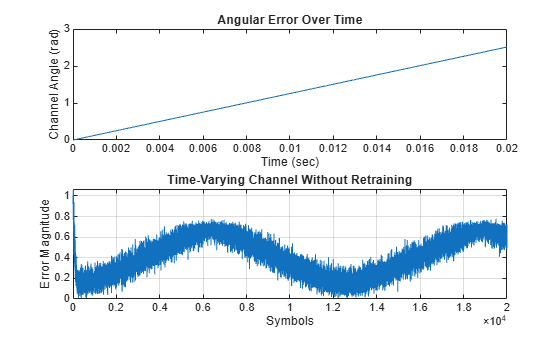`scatterplot(y)`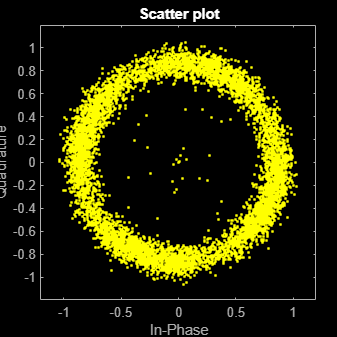Set the `TrainingInputPort` property to `true` to configure the equalizer to retrain the taps when signaled by the `trainFlag` input. The equalizer trains only when `trainFlag` is `true`. After every 2000 symbols, the equalizer retrains the taps and keeps lock on variations of the channel. Plot the angular error from the channel, the equalizer error signal, and signal constellation. As the channel varies, the equalizer output removes the channel effects. Also, the output constellation does not rotate out of sync, and bit errors are reduced.

```release(dfeq) dfeq.TrainingFlagInputPort = true; symbolCnt = 0; numPackets = length(rx)/spp; trainFlag = true; trainingPeriod = 2000; eVec = zeros(size(rx)); yVec = zeros(size(rx)); for p=1:numPackets [yVec((p-1)*spp+1:p*spp,1),eVec((p-1)*spp+1:p*spp,1)] = ... dfeq(rx((p-1)*spp+1:p*spp,1), ... trainingSymbols,trainFlag); symbolCnt = symbolCnt + spp; if symbolCnt >= trainingPeriod trainFlag = true; symbolCnt = 0; else trainFlag = false; end end figure subplot(2,1,1) plot(tx, unwrap(angle(channel))) xlabel('t (sec)') ylabel('Channel Angle (rad)') title('Angular Error Over Time') subplot(2,1,2) plot(abs(eVec)) xlabel('Symbols') ylabel('Error Magnitude') grid on title('Time-Varying Channel With Retraining')````scatterplot(yVec)`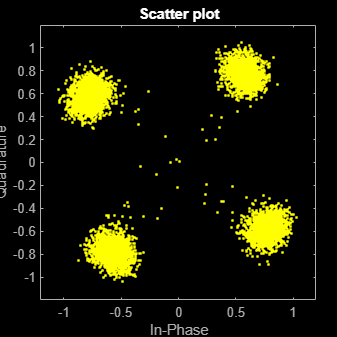Simulate a system with delay between the transmitted symbols and received samples. Typical systems have transmitter and receiver filters that result in a delay. This delay must be accounted for to synchronize the system. In this example, the system delay is introduced without transmit and receive filters. Decision feedback equalization, using the least mean squares (LMS) algorithm, recovers QPSK symbols.

Initialize simulation variables.

```M = 4; % QPSK numSymbols = 10000; numTrainingSymbols = 1000; mpChan = [1 0.5*exp(1i*pi/6) 0.1*exp(-1i*pi/8)]; systemDelay = dsp.Delay(20); snr = 24;```

Generate QPSK-modulated symbols. Apply multipath channel filtering, a system delay, and AWGN to the transmitted symbols.

```data = randi([0 M-1],numSymbols,1); tx = pskmod(data,M,pi/4); % OQPSK delayedSym = systemDelay(filter(mpChan,1,tx)); rx = awgn(delayedSym,snr,'measured');```

Create equalizer and EVM System objects. The equalizer System object specifies a decision feedback equalizer using the LMS algorithm.

```dfeq = comm.DecisionFeedbackEqualizer('Algorithm','LMS', ... 'NumForwardTaps',9,'NumFeedbackTaps',6,'ReferenceTap',5); evm = comm.EVM('ReferenceSignalSource', ... 'Estimated from reference constellation');```

`[y1,err1,wts1] = dfeq(rx,tx(1:numTrainingSymbols,1));`

Find the delay between the received symbols and the transmitted symbols by using the `finddelay` function.

`rxDelay = finddelay(tx,rx)`
```rxDelay = 20 ```

Display the equalizer information. The latency value indicates the delay introduced by the equalizer. Calculate the total delay as the sum of `rxDelay` and the equalizer latency.

`eqInfo = info(dfeq)`
```eqInfo = struct with fields: Latency: 4 ```
`totalDelay = rxDelay + eqInfo.Latency;`

Until the equalizer output converges, the symbol error rate is high. Plot the error output, `err1`, to determine when the equalized output converges.

```plot(abs(err1)) xlabel('Symbols') ylabel('Error Magnitude') title('Equalizer Error Signal')```The plot shows excessive errors for the first 2000 symbols. When demodulating symbols and computing symbol errors, account for the unconverged output and the system delay between the equalizer output and transmitted symbols.

```dataRec1 = pskdemod(y1(2000+totalDelay:end),M,pi/4); symErrWithDelay = symerr(data(2000:end-totalDelay),dataRec1)```
```symErrWithDelay = 5977 ```
`evmWithDelay = evm(y1)`
```evmWithDelay = 26.3288 ```

The error rate and EVM are high because the receive delay was not accounted for in the equalizer System object.

Adjust Input Delay in Decision Feedback Equalizer

Equalize the received data by using the delay value to set the `InputDelay` property. Since `InputDelay` is a nontunable property, you must release the `dfeq` System object to reconfigure the `InputDelay` property. Equalize the received symbols.

```release(dfeq) dfeq.InputDelay = rxDelay```
```dfeq = comm.DecisionFeedbackEqualizer with properties: Algorithm: 'LMS' NumForwardTaps: 9 NumFeedbackTaps: 6 StepSize: 0.0100 Constellation: [0.7071 + 0.7071i -0.7071 + 0.7071i -0.7071 - 0.7071i 0.7071 - 0.7071i] ReferenceTap: 5 InputDelay: 20 InputSamplesPerSymbol: 1 TrainingFlagInputPort: false AdaptAfterTraining: true InitialWeightsSource: 'Auto' WeightUpdatePeriod: 1 ```
`[y2,err2,wts2] = dfeq(rx,tx(1:numTrainingSymbols,1));`

Plot the tap weights and equalized error magnitude. A stem plot shows the equalizer tap weights before and after the system delay is removed. A 2-D line plot shows the slower equalizer convergence for the delayed signal, as compared to the signal with the delay removed.

```subplot(2,1,1) stem([real(wts1),real(wts2)]) xlabel('Taps') ylabel('Tap Weight Real') legend('rxDelayed','rxDelayRemoved') grid on subplot(2,1,2) stem([imag(wts1),imag(wts2)]) xlabel('Taps') ylabel('Tap Weight Imaginary') legend('rxDelayed','rxDelayRemoved') grid on```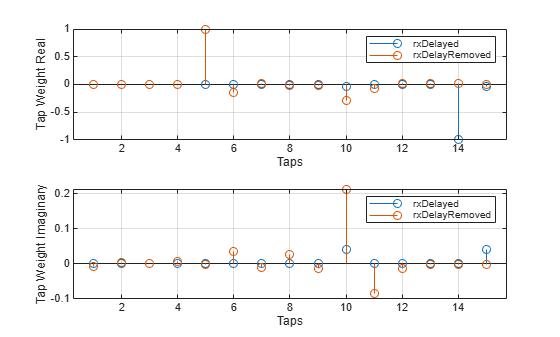```figure plot([abs(err1),abs(err2)]) xlabel('Symbols') ylabel('Error Magnitude') legend('rxDelayed','rxDelayRemoved') grid on```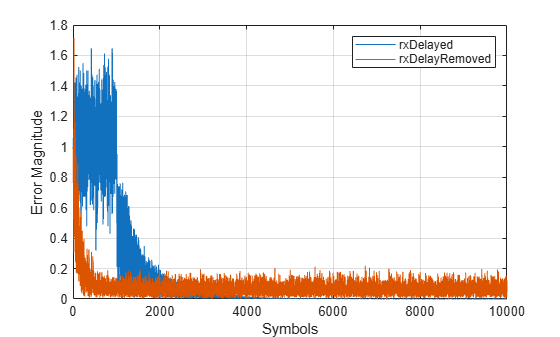Plot error output of the equalized signals, `rxDelayed` and `rxDelayRemoved`. For the signal that has the delay removed, the equalizer converges during the 1000 symbol training period. When demodulating symbols and computing symbol errors, to account for the unconverged output and the system delay between the equalizer output and transmitted symbols, skip the first 500 symbols. Reconfiguring the equalizer to account for the system delay enables better equalization of the signal, and reduces symbol errors and the EVM.

`eqInfo = info(dfeq)`
```eqInfo = struct with fields: Latency: 4 ```
```totalDelay = rxDelay + eqInfo.Latency; dataRec2 = pskdemod(y2(500+totalDelay:end),M,pi/4); symErrDelayRemoved = symerr(data(500:end-totalDelay),dataRec2)```
```symErrDelayRemoved = 0 ```
`evmDelayRemoved = evm(y2(500+totalDelay:end))`
```evmDelayRemoved = 7.5200 ```

Recover QPSK symbols with a decision equalizer, using the constant modulus algorithm (CMA) and EVM-based taps training. When using blind equalizer algorithms, such as CMA, you can train the equalizer taps using the `AdaptWeights` property to start and stop training. Use helper functions to generate plots and apply phase correction.

Initialize system variables.

```rng(123456); M = 4; % QPSK numSymbols = 100; numPackets = 5000; refTap = 3; nFwdTaps = 5; nFdbkTaps = 4; ttlTaps = nFwdTaps + nFdbkTaps; raylChan = comm.RayleighChannel( ... 'PathDelays',[0 1], ... 'AveragePathGains',[0 -12], ... 'MaximumDopplerShift',1e-5); SNR = 50; adaptWeights = true;```

Create the equalizer and EVM System objects. The equalizer System object specifies a decision feedback equalizer using the CMA adaptive algorithm. Call the helper function to initialize figure plots.

```dfeq = comm.DecisionFeedbackEqualizer( ... 'Algorithm','CMA', ... 'NumForwardTaps',nFwdTaps, ... 'NumFeedbackTaps',nFdbkTaps, ... 'ReferenceTap',refTap, ... 'StepSize',0.03, ... 'AdaptWeightsSource','Input port')```
```dfeq = comm.DecisionFeedbackEqualizer with properties: Algorithm: 'CMA' NumForwardTaps: 5 NumFeedbackTaps: 4 StepSize: 0.0300 Constellation: [0.7071 + 0.7071i -0.7071 + 0.7071i -0.7071 - 0.7071i 0.7071 - 0.7071i] ReferenceTap: 3 InputSamplesPerSymbol: 1 AdaptWeightsSource: 'Input port' InitialWeightsSource: 'Auto' WeightUpdatePeriod: 1 ```
`info(dfeq)`
```ans = struct with fields: Latency: 2 ```
```evm = comm.EVM('ReferenceSignalSource', ... 'Estimated from reference constellation'); [errPlot,evmPlot,scatSym,adaptState] = ... initFigures(numPackets,ttlTaps);```

Equalization Loop

Follow these steps to implement the equalization loop.

1. Generate OQPSK data packets.

2. Apply Rayleigh fading and AWGN to the transmission data.

3. Apply equalization to the received data and phase correction to the equalizer output.

4. Estimate the EVM and toggle the `adaptWeights` flag to `true` or `false` based on the EVM level.

5. Update the figure plots.

```for p=1:numPackets data = randi([0 M-1],numSymbols,1); tx = pskmod(data,M,pi/4); rx = awgn(raylChan(tx),SNR); rxDelay = finddelay(rx,tx); [y,err,wts] = dfeq(rx,adaptWeights); y = phaseCorrection(y); evmEst = evm(y); adaptWeights = (evmEst > 20); updateFigures(errPlot,evmPlot,scatSym,adaptState, ... wts,y(end),evmEst,adaptWeights,p,numPackets) end```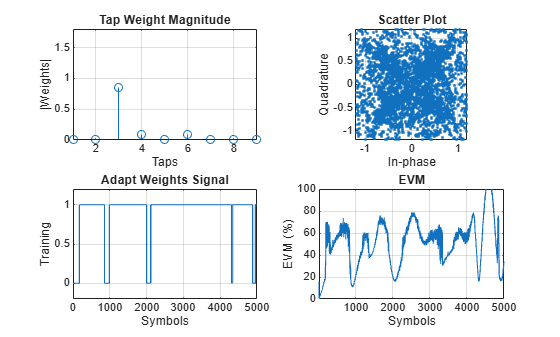`rxDelay`
```rxDelay = 0 ```

The figure plots show that, as the EVM varies, the equalizer toggles in and out of decision-directed weight adaptation mode.

Helper Functions

This helper function initializes figures that show a quad plot of simulation results.

```function [errPlot,evmPlot,scatter,adaptState] = ... initFigures(numPkts,ttlTaps) yVec = nan(numPkts,1); evmVec = nan(numPkts,1); wVec = zeros(ttlTaps,1); adaptVec = nan(numPkts,1); figure subplot(2,2,1) evmPlot = stem(wVec); grid on; axis([1 ttlTaps 0 1.8]) xlabel('Taps'); ylabel('|Weights|'); title('Tap Weight Magnitude') subplot(2,2,2) scatter = plot(yVec, '.'); axis square; axis([-1.2 1.2 -1.2 1.2]); grid on; xlabel('In-phase'); ylabel('Quadrature'); title('Scatter Plot'); subplot(2,2,3) adaptState = plot(adaptVec); grid on; axis([0 numPkts -0.2 1.2]) ylabel('Training'); xlabel('Symbols'); title('Adapt Weights Signal') subplot(2,2,4) errPlot = plot(evmVec); grid on; axis([1 numPkts 0 100]) xlabel('Symbols'); ylabel('EVM (%)'); title('EVM') end ```

This helper function updates the figures.

```function updateFigures(errPlot,evmPlot,scatSym, ... adaptState,w,y,evmEst,adaptWts,p,numFrames) persistent yVec evmVec adaptVec if p == 1 yVec = nan(numFrames,1); evmVec = nan(numFrames,1); adaptVec = nan(numFrames,1); end yVec(p) = y; evmVec(p) = evmEst; adaptVec(p) = adaptWts; errPlot.YData = abs(evmVec); evmPlot.YData = abs(w); scatSym.XData = real(yVec); scatSym.YData = imag(yVec); adaptState.YData = adaptVec; drawnow limitrate end ```

This helper function applies phase correction.

```function y = phaseCorrection(y) a = angle(y((real(y) > 0) & (imag(y) > 0))); a(a < 0.1) = a(a < 0.1) + pi/2; theta = mean(a) - pi/4; y = y * exp(-1i*theta); end```

Recover QPSK symbols in fading environments with a decision feedback equalizer, using the least mean squares (LMS) algorithm. Use the `reset` object function to equalize independent packets. Use helper functions to generate plots. This example also shows symbol-based processing and frame-based processing.

Setup

Initialize system variables, create the equalizer System object, and initialize the plot figures.

```M = 4; % QPSK numSym = 1000; numTrainingSym = 100; numPackets = 5; refTap = 5; nFwdTaps = 9; nFdbkTaps = 4; ttlTaps = nFwdTaps + nFdbkTaps; stepsz = 0.01; ttlNumSym = numSym + numTrainingSym; raylChan = comm.RayleighChannel( ... 'PathDelays',[0 1], ... 'AveragePathGains',[0 -9], ... 'MaximumDopplerShift',0, ... 'PathGainsOutputPort',true); SNR = 35; rxVec = zeros(ttlNumSym,numPackets); txVec = zeros(ttlNumSym,numPackets); yVec = zeros(ttlNumSym,1); eVec = zeros(ttlNumSym,1); dfeq1 = comm.DecisionFeedbackEqualizer( ... 'Algorithm','LMS', ... 'NumForwardTaps',nFwdTaps, ... 'NumFeedbackTaps',nFdbkTaps, ... 'ReferenceTap',refTap, ... 'StepSize',stepsz, ... 'TrainingFlagInputPort',true); [errPlot,wStem,hStem,scatPlot] = ... initFigures(ttlNumSym,ttlTaps, ... raylChan.AveragePathGains);```

Symbol-Based Processing

For symbol-based processing, provide one symbol at the input of the equalizer. Reset the equalizer state and channel after processing each packet.

```for p = 1:numPackets trainingFlag = true; for q=1:ttlNumSym data = randi([0 M-1],1,1); tx = pskmod(data,M,pi/4); [xc,pg] = raylChan(tx); rx = awgn(xc,25); [y,err,wts] = dfeq1(rx,tx,trainingFlag);```

Disable training after processing `numTrainingSym` training symbols.

``` if q == numTrainingSym trainingFlag = false; end updateFigures(errPlot,wStem,hStem, ... scatPlot,err,wts,y,pg,q,ttlNumSym); txVec(q,p) = tx; rxVec(q,p) = rx; end```

After processing each packet, reset the channel System object to get a new realization of channel taps and the equalizer System object to restore the default taps weights.

``` reset(raylChan) reset(dfeq1) end```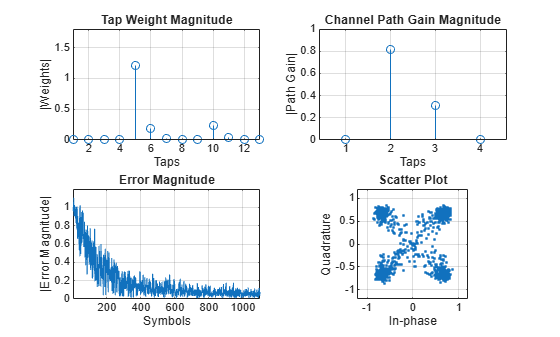Packet-Based Processing

For packet-based processing, provide one packet at the input of the equalizer. Each packet contains `ttlNumSym` symbols. Because the training duration is less than the packet length, you do not need to specify the start-training input.

```yVecPkt = zeros(ttlNumSym,numPackets); errVecPkt = zeros(ttlNumSym,numPackets); wgtVecPkt = zeros(ttlTaps,numPackets); dfeq2 = comm.DecisionFeedbackEqualizer( ... 'Algorithm','LMS', ... 'NumForwardTaps',nFwdTaps, ... 'NumFeedbackTaps',nFdbkTaps, ... 'ReferenceTap',refTap, ... 'StepSize',stepsz); for p = 1:numPackets [yVecPkt(:,p),errVecPkt(:,p),wgtVecPkt(:,p)] = ... dfeq2(rxVec(:,p),txVec(1:numTrainingSym,p)); for q=1:ttlNumSym updateFigures(errPlot,wStem,hStem,scatPlot, ... errVecPkt(q,p),wgtVecPkt(:,p),yVecPkt(q,p), ... pg,q,ttlNumSym); end```

After processing each packet, reset the channel System object to get a new realization of channel taps and the equalizer System object to restore the default taps weights.

``` reset(raylChan) reset(dfeq2) end```Helper Functions

This helper function initializes the figures.

```function [errPlot,wStem,hStem,scatPlot] = ... initFigures(ttlNumSym,ttlTap,pg) yVec = nan(ttlNumSym,1); eVec = nan(ttlNumSym,1); wVec = zeros(ttlTap,1); figure; subplot(2,2,1); wStem = stem(wVec); axis([1 ttlTap 0 1.8]); grid on; xlabel('Taps'); ylabel('|Weights|'); title('Tap Weight Magnitude') subplot(2,2,2); hStem = stem([0 abs(pg) 0]); grid on; xlabel('Taps'); ylabel('|Path Gain|'); title('Channel Path Gain Magnitude') subplot(2,2,3); errPlot = plot(eVec); axis([1 ttlNumSym 0 1.2]); grid on xlabel('Symbols'); ylabel('|Error Magnitude|'); title('Error Magnitude') subplot(2,2,4); scatPlot = plot(yVec,'.'); axis square; axis([-1.2 1.2 -1.2 1.2]); grid on; xlabel('In-phase'); ylabel('Quadrature'); title(sprintf('Scatter Plot')); end```

This helper function updates the figures.

```function updateFigures(errPlot,wStem,hStem,scatPlot, ... err,wts,y,pg,p,ttlNumSym) persistent yVec eVec if p == 1 yVec = nan(ttlNumSym,1); eVec = nan(ttlNumSym,1); end yVec(p) = y; eVec(p) = abs(err); errPlot.YData = abs(eVec); wStem.YData = abs(wts); hStem.YData = [0 abs(pg) 0]; scatPlot.XData = real(yVec); scatPlot.YData = imag(yVec); drawnow limitrate end```

expand all

expand all

## Version History

Introduced in R2019a